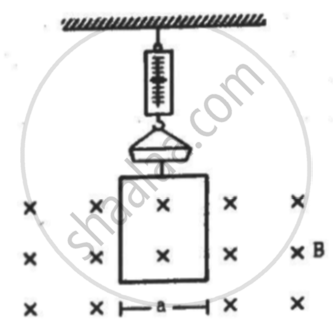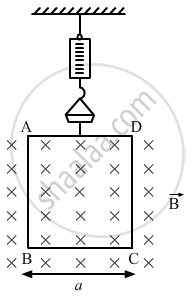Advertisement Remove all ads

# A Rectangular Wire-loop of Width a is Suspended from the Insulated Pan of a Spring Balance, as Shown in the Figure. a Current I Exists in the Anti-clockwise Direction - Physics

Sum

A rectangular wire-loop of width a is suspended from the insulated pan of a spring balance, as shown in the figure. A current exists in the anti-clockwise direction in the loop. A magnetic field B exists in the lower region. Find the change in the tension of the spring if the current in the loop is reversed.Advertisement Remove all ads

#### Solution

Given,
A rectangular wire loop of width a
Electric current through the loop = i
Direction of the current is anti-clockwise.
Strength of the magnetic field in the lower region = B
Direction of the magnetic field is into the plane of the loop.Here, angle between the length of the loop and magnetic field, θ = 90˚
Magnetic force is given by
vecF = i vecaxxvecB
The magnetic force will act only on side AD and BC.
As side AD is outside the magnetic field, so F = 0
Magnetic force on side BC is
vecF = i veca xx vecB
= iaBsin theta
= iaB
Direction of force can be found using Fleming's left-hand rule.
Thus, the direction of the magnetic force is upward.
Similarly if we change the direction of current to clockwise,
the force along BC,
vecF = i veca xx vecB

Thus, the change in force is equal to the change in tension
iaB − (− iaB) = 2iaB.

Concept: Torque on a Current Loop in Magnetic Field
Is there an error in this question or solution?
Advertisement Remove all ads

#### APPEARS IN

HC Verma Class 11, Class 12 Concepts of Physics Vol. 2
Chapter 12 Magnetic Field
Q 14 | Page 231
Advertisement Remove all ads

#### Video TutorialsVIEW ALL 

Advertisement Remove all ads
Share
Notifications

View all notifications

Forgot password?